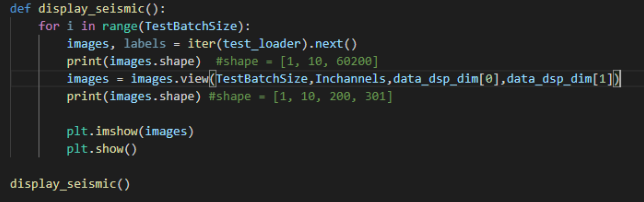# Plot 4D tensor as image

Hi All, I’m trying to plot a randomly sampled image from my test data as shown in the code below but I get this error `TypeError: Invalid dimensions for image data`. The image tensor is a 4D tensor with shape (TestBatchSIze, Channels, height, width). Any ideas to help resolve this error and plot the data are welcomed.`plt.imshow` expects a numpy array in the shape `[height, width, channels]`.
Given your input has the shape `[batch_size, channels, height, width]` this should work:

``````batch_size, channels, height, width = 2, 3, 224, 224
x = torch.randn(batch_size, channels, height, width)

# select a sample from the batch
img = x
# permute to match the desired memory format
img = img.permute(1, 2, 0).numpy()
plt.imshow(img)
``````

@ptrblck. The no. of channels in my image tensor is 10, hence when I use permute I still get the same error. I guess permute expects the no. of channels to be 3 or 4. Is there a way to plot if the no. of channels is more than 4?

`permute` will work on any number of channels, as it’s only permuting the dimensions.
`plt.imshow` expects an “image format”, i.e. a numpy array with either 3 channels (RGB), a single channel (grayscale), or no channel dimension (also grayscale or an arbitrary matrix which will be visualized using the specified colormap).
10 channels do not represent a valid image format so you won’t be able to visualize it directly.
I don’t know what this data represents, but you might want to create 10 subplots, where each one visualizes a single channel.